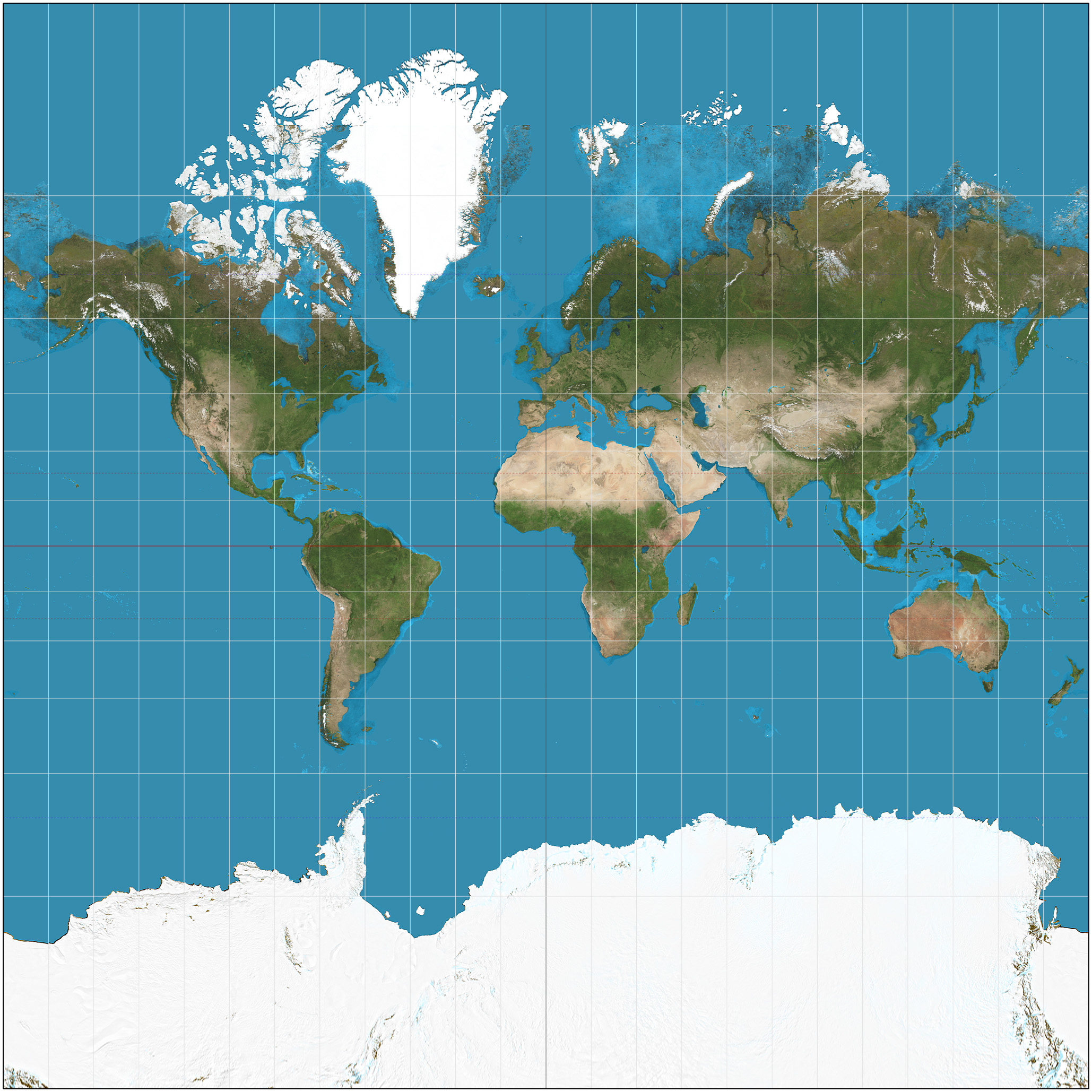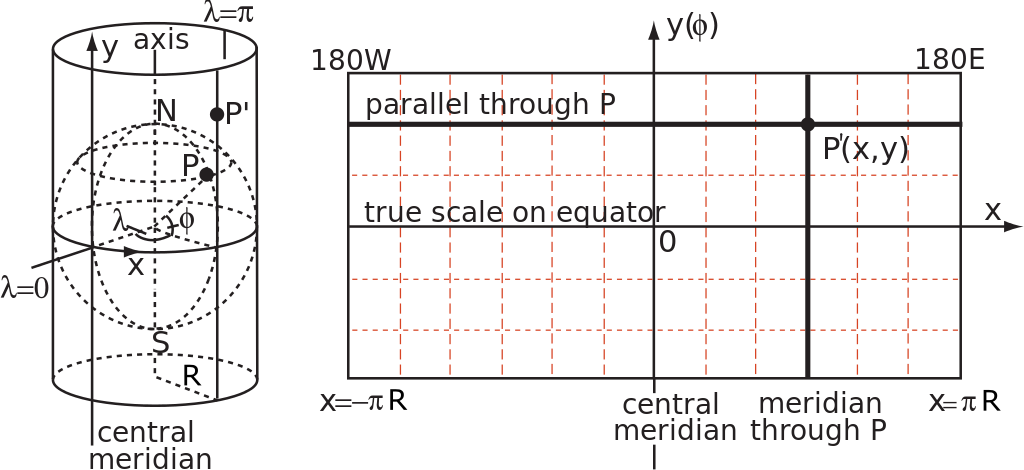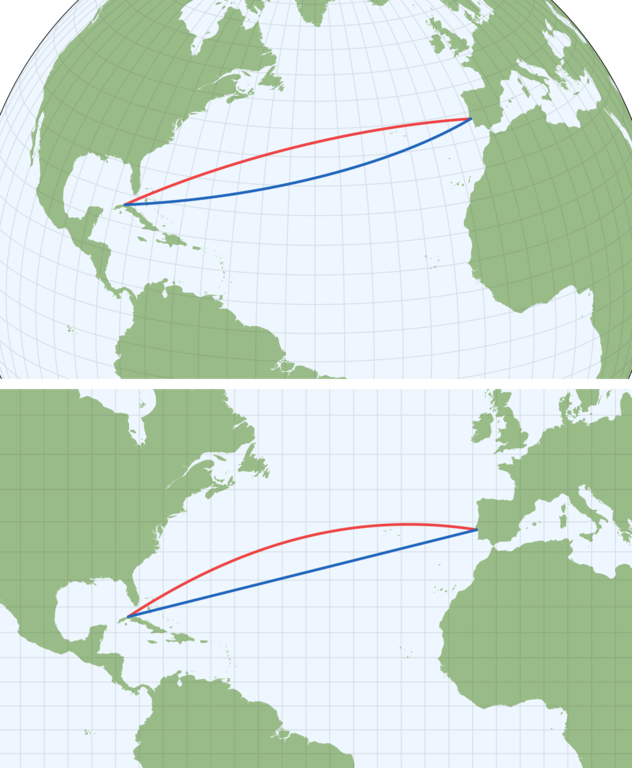By Long Luo

# 如何绘制地图？# 什么是墨卡托投影？# 墨卡托公式的推导

$P(lng, lat)$ 表示投影前的球面上的点，在数学上我们常使用弧度制，则表示为 $P(\lambda , \varphi )$ ，其中 $\lambda = lng \cdot \frac{\pi}{180}$$\varphi = lat \cdot \frac{\pi}{180}$ ，所以墨卡托投影就是将球面上的 $P(\lambda , \varphi )$ 转换为平面直角坐标系 $P' (x, y)$ ，即定义为下面的一个映射：

$f: P \rightarrow P'$

\left\{ \begin{aligned} x &= f_x(\lambda , \varphi ) \\ y &= f_y(\lambda , \varphi ) \\ \end{aligned} \right.$P$ 的经纬度为 $[\lambda , \varphi ]$ ，相邻点 $Q$ 的经纬度为 $[\lambda + \mathrm{d}\lambda , \varphi + \mathrm{d}\varphi]$ ，那么平行赤道的纬线所在圆的半径 $r = R \cos \varphi$ ，则有圆弧：

$PM = r \times \mathrm{d} \lambda = R \cos \varphi \mathrm{d} \lambda$

，圆弧 $PK = R \mathrm{d} \varphi$ 。投影变换后平面上的矩形长宽分别为 $\mathrm{d} x$$\mathrm{d} y$

$\frac{R \cdot \mathrm{d} {\varphi}}{\mathrm{d} y} = \frac{R \cdot cos \varphi \cdot \mathrm{d} \lambda }{\mathrm{d} x}$

$tan \angle \alpha \approx \frac{PM}{QM} = \frac{R \cos \varphi \mathrm{d} \lambda }{R \mathrm{d} \varphi }$

$tan \angle \beta = \frac{P'M'}{Q'M'} = \frac{\mathrm{d} x}{\mathrm{d} y}$

$\frac{R \cos \varphi \mathrm{d} \lambda }{R \mathrm{d} \varphi } = \frac{\mathrm{d} x}{\mathrm{d} y}$

\left \{ \begin{aligned} x'(\lambda ) &= R \\ y'(\varphi ) &=R \cdot \frac{1}{\cos \varphi } \cdot \mathrm{d} {\varphi} = R \sec \varphi \\ \end{aligned} \right.

$\int dy = R \int \frac{1}{ \cos \varphi} d\varphi = R \int \sec \varphi d \varphi = R \ln(\sec \varphi + \tan \varphi ) + C$

$y = R \ln \tan(\frac{\pi}{4} + \frac{\varphi }{2}) + C$

\left \{ \begin{aligned} x &= R(\lambda -\lambda _{0}) \\ y &= R\ln \left[\tan \left({\frac {\pi }{4}} + {\frac {\varphi }{2}}\right)\right] \\ \end{aligned} \right.

# 等角航线